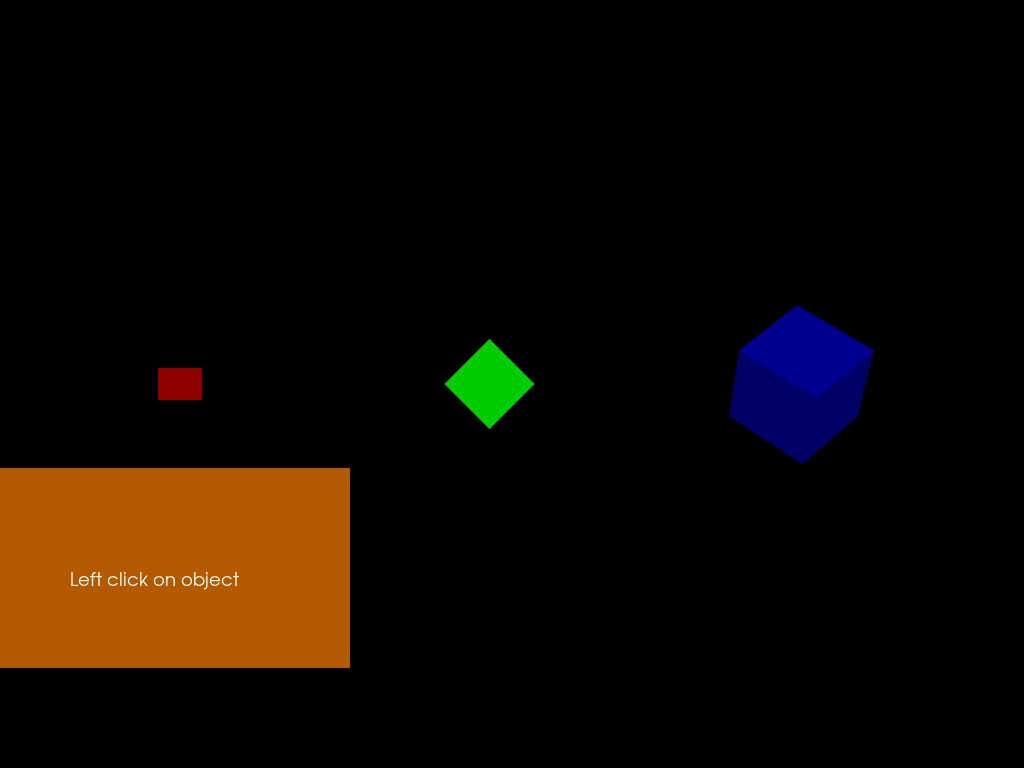# Simple picking#

Here we present a tutorial showing how to interact with objects in the 3D world. All objects to be picked are part of a single actor. FURY likes to bundle objects in a few actors to reduce code and increase speed.

When the objects are picked they will change size and color.

```import numpy as np

from fury import actor, pick, ui, utils, window

centers = 0.5 * np.array([[0, 0, 0], [100, 0, 0], [200, 0, 0.0]])
colors = np.array([[0.8, 0, 0], [0, 0.8, 0], [0, 0, 0.8]])
radii = 0.1 * np.array([50, 100, 150.0])

selected = np.zeros(3, dtype=bool)
```

Let’s create a panel to show what is picked

```panel = ui.Panel2D(size=(400, 200), color=(1, 0.5, 0.0), align='right')
panel.center = (150, 200)

text_block = ui.TextBlock2D(text='Left click on object \n')
```

Build scene and add an actor with many objects.

```scene = window.Scene()

label_actor = actor.vector_text(text='Test')
```

This actor is made with 3 cubes of different orientation

```directions = np.array(
[
[np.sqrt(2) / 2, 0, np.sqrt(2) / 2],
[np.sqrt(2) / 2, np.sqrt(2) / 2, 0],
[0, np.sqrt(2) / 2, np.sqrt(2) / 2],
]
)
fury_actor = actor.cube(centers, directions, colors, scales=radii)
```

Access the memory of the vertices of all the cubes

```vertices = utils.vertices_from_actor(fury_actor)
num_vertices = vertices.shape
num_objects = centers.shape
```

Access the memory of the colors of all the cubes

```vcolors = utils.colors_from_actor(fury_actor, 'colors')
```

Adding an actor showing the axes of the world coordinates

```ax = actor.axes(scale=(10, 10, 10))

scene.reset_camera()
```

Create the Picking manager

```pickm = pick.PickingManager()
```

Time to make the callback which will be called when we pick an object

```def left_click_callback(obj, event):

# Get the event position on display and pick

event_pos = pickm.event_position(showm.iren)
picked_info = pickm.pick(event_pos, showm.scene)

vertex_index = picked_info['vertex']

# Calculate the objects index

object_index = int(np.floor((vertex_index / num_vertices) * num_objects))

# Find how many vertices correspond to each object
sec = int(num_vertices / num_objects)

if not selected[object_index]:
scale = 6 / 5
color_add = np.array([30, 30, 30], dtype='uint8')
selected[object_index] = True
else:
scale = 5 / 6
color_add = np.array([-30, -30, -30], dtype='uint8')
selected[object_index] = False

# Update vertices positions
vertices[object_index * sec : object_index * sec + sec] = (
scale
* (
vertices[object_index * sec : object_index * sec + sec]
- centers[object_index]
)
+ centers[object_index]
)

# Update colors
vcolors[object_index * sec : object_index * sec + sec] += color_add

# Tell actor that memory is modified
utils.update_actor(fury_actor)

face_index = picked_info['face']

# Show some info
text = 'Object ' + str(object_index) + '\n'
text += 'Vertex ID ' + str(vertex_index) + '\n'
text += 'Face ID ' + str(face_index) + '\n'
text += 'World pos ' + str(np.round(picked_info['xyz'], 2)) + '\n'
text += 'Actor ID ' + str(id(picked_info['actor']))
text_block.message = text
showm.render()
```

Bind the callback to the actor

```fury_actor.AddObserver('LeftButtonPressEvent', left_click_callback, 1)
```
```1
```

Make the window appear

```showm = window.ShowManager(scene, size=(1024, 768), order_transparent=True)

```

Change interactive to True to start interacting with the scene

```interactive = False

if interactive:

showm.start()
```

Save the current framebuffer in a PNG file

```window.record(showm.scene, size=(1024, 768), out_path='viz_picking.png')
```Total running time of the script: ( 0 minutes 0.116 seconds)

Gallery generated by Sphinx-Gallery